Lines of Symmetry Math Video for Kids | 3rd, 4th, & 5th Grade
1%
It was processed successfully!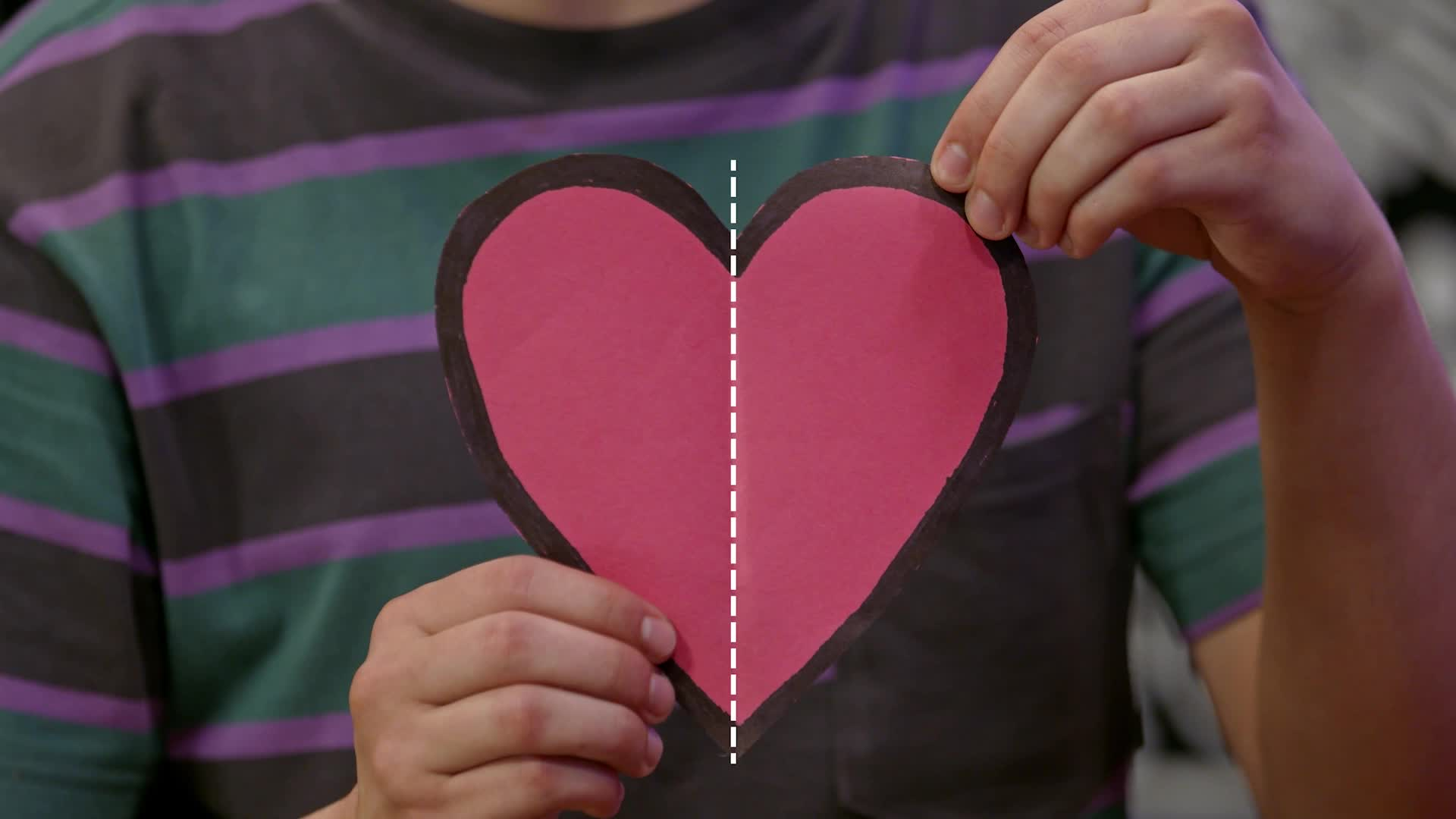WHAT ARE LINES OF SYMMETRY?

A shape has symmetry if it can be divided by a line so that each half is a mirror image of the other. A line of symmetry is a line that divides a shape in that way.

To better understand lines of symmetry…

WHAT ARE LINES OF SYMMETRY?. A shape has symmetry if it can be divided by a line so that each half is a mirror image of the other. A line of symmetry is a line that divides a shape in that way. To better understand lines of symmetry…

## LET’S BREAK IT DOWN!

### Faces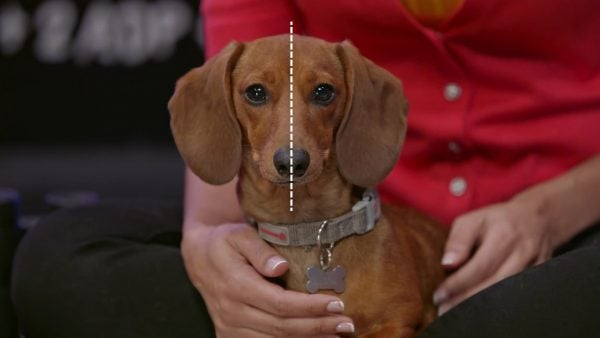Adesina has a red paper heart. Marcos folds the paper heart in half and April notices that the two sides match. We learn that a shape has symmetry if it can be divided by a line so that each half is a mirror image of the other. The line that divides the shape is called the line of symmetry. If we draw a line down the middle (from top to bottom) of a person’s or animal’s face, we can see that faces are mostly symmetrical too. If we try to split a face in half by drawing a line from left to right, we see that the two halves do not match: they are not mirror images. So, the line drawn from top to bottom is a line of symmetry, but the line drawn from left to right is not a line of symmetry. If we draw a line dividing an image of a butterfly into a left half and a right half, both sides are mirror images, so the line is a line of symmetry. If we draw the line across, the top half does not match the bottom half: the top has antennas but the bottom does not. Then the line across is not a line of symmetry. Some objects have many lines of symmetry. A 3-leaf clover has 3 lines of symmetry. Now you try: Can you think of something in the real world that has two lines of symmetry?

Faces Adesina has a red paper heart. Marcos folds the paper heart in half and April notices that the two sides match. We learn that a shape has symmetry if it can be divided by a line so that each half is a mirror image of the other. The line that divides the shape is called the line of symmetry. If we draw a line down the middle (from top to bottom) of a person’s or animal’s face, we can see that faces are mostly symmetrical too. If we try to split a face in half by drawing a line from left to right, we see that the two halves do not match: they are not mirror images. So, the line drawn from top to bottom is a line of symmetry, but the line drawn from left to right is not a line of symmetry. If we draw a line dividing an image of a butterfly into a left half and a right half, both sides are mirror images, so the line is a line of symmetry. If we draw the line across, the top half does not match the bottom half: the top has antennas but the bottom does not. Then the line across is not a line of symmetry. Some objects have many lines of symmetry. A 3-leaf clover has 3 lines of symmetry. Now you try: Can you think of something in the real world that has two lines of symmetry?

### Symmetry in Shapes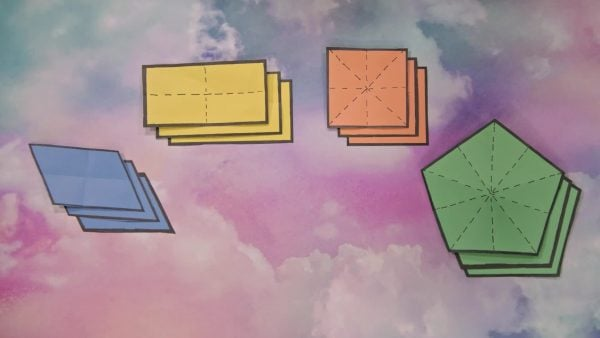Adesina folds a cut-out rectangle in half from top to bottom and the edges of the two halves match up perfectly. This means that the fold is a line of symmetry. She unfolds the rectangle and then folds it from left to right and the edges of the two halves match up again. This rectangle has two lines of symmetry. When she folds the rectangle diagonally, the edges of the two halves do not match up. The diagonal line is not a line of symmetry on a rectangle. We discover that a square has two diagonal lines of symmetry, as well as the folds from top to bottom and left to right. We discover that a parallelogram does not have symmetry because the edges do not match up, no matter how we fold it. Only parallelograms that are rectangles have symmetry, and only parallelograms that are squares have 4 lines of symmetry. Now you try: What is a letter of the alphabet that has no lines of symmetry?

Symmetry in Shapes Adesina folds a cut-out rectangle in half from top to bottom and the edges of the two halves match up perfectly. This means that the fold is a line of symmetry. She unfolds the rectangle and then folds it from left to right and the edges of the two halves match up again. This rectangle has two lines of symmetry. When she folds the rectangle diagonally, the edges of the two halves do not match up. The diagonal line is not a line of symmetry on a rectangle. We discover that a square has two diagonal lines of symmetry, as well as the folds from top to bottom and left to right. We discover that a parallelogram does not have symmetry because the edges do not match up, no matter how we fold it. Only parallelograms that are rectangles have symmetry, and only parallelograms that are squares have 4 lines of symmetry. Now you try: What is a letter of the alphabet that has no lines of symmetry?

### Drawing Using Lines of Symmetry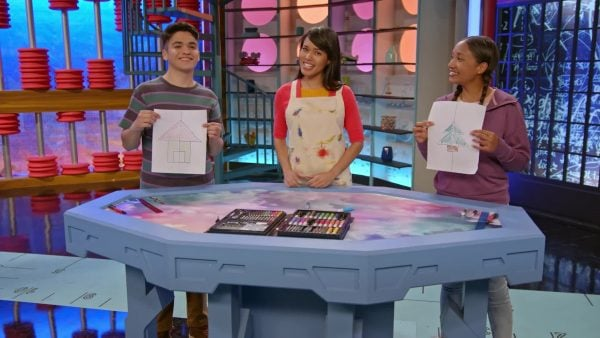Take a piece of grid paper and fold it along one of the grid lines. Now, think of something simple to draw using straight lines, and draw exactly half of it on one side of the folded line. Color it in, and switch drawings with a partner. Finish their image by drawing the mirror image on the other side of the fold line. April draws a house and notices that if a line is 4 grid lines to the left of the fold on one side, its mirror image will be exactly 4 grid lines to the right of the fold on the other side. Every part of the drawing on the left side must be the same distance from the fold as the matching parts of the drawing on the right side. Lastly, we learn that a circle has an infinite number of lines of symmetry if it is folded exactly through the middle. Now you try: Do the drawing activity on your own. Fold a piece of grid paper along a line, and think of a simple image to draw. Draw half of it on one side of the line, and then complete the drawing by creating the mirror image on the other side. Do they match?

Drawing Using Lines of Symmetry Take a piece of grid paper and fold it along one of the grid lines. Now, think of something simple to draw using straight lines, and draw exactly half of it on one side of the folded line. Color it in, and switch drawings with a partner. Finish their image by drawing the mirror image on the other side of the fold line. April draws a house and notices that if a line is 4 grid lines to the left of the fold on one side, its mirror image will be exactly 4 grid lines to the right of the fold on the other side. Every part of the drawing on the left side must be the same distance from the fold as the matching parts of the drawing on the right side. Lastly, we learn that a circle has an infinite number of lines of symmetry if it is folded exactly through the middle. Now you try: Do the drawing activity on your own. Fold a piece of grid paper along a line, and think of a simple image to draw. Draw half of it on one side of the line, and then complete the drawing by creating the mirror image on the other side. Do they match?

## LINES OF SYMMETRY VOCABULARY

Mirror Image
A flipped version of an image, like you would see on the other side of a mirror.
Symmetry
A shape has symmetry if it can be divided by a line so that each half is a mirror image of the other.
Line of Symmetry
The line that divides a shape into two mirror images.
Rectangle
A 4-sided shape that has two sets of parallel lines that connect at right angles.
Square
A type of rectangle with 4 sides of equal length.
Parallelogram
A 4-sided shape that has two sets of parallel lines.
Connecting two opposite corners.
Going on forever; more than we could ever count.

## LINES OF SYMMETRY DISCUSSION QUESTIONS

### Does every object have a line of symmetry?

No. Some objects have no lines of symmetry, some have 1 line, and some have more.

### What are some letters of the alphabet that have lines of symmetry? Do any have more than one?

If letters are written very simply, then these have 1 line of symmetry: A, B, C, D, E, M.
These have 2 lines of symmetry: I, O, H, X.
These have none: F, G, J, L, N.

### Is it true that if we fold a circle anywhere, the fold will be a line of symmetry?

No. A circle has an infinite number of lines of symmetry, but the line must go through the center of the circle. So the circle must be folded in half.
X

## Success

We’ve sent you an email with instructions how to reset your password.
Ok

## Choose Your Free Trial Period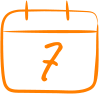### 7 DaysContinue to Lessons### 90 DaysGet 90 days free by inviting other teachers to try it too.

Share with Teachers

## Get 90 Days Free

### By inviting 4 other teachers to try it too.

4 required

Skip, I will use a 7 days free trial

## Thank You!

Enjoy your free 90 days trial